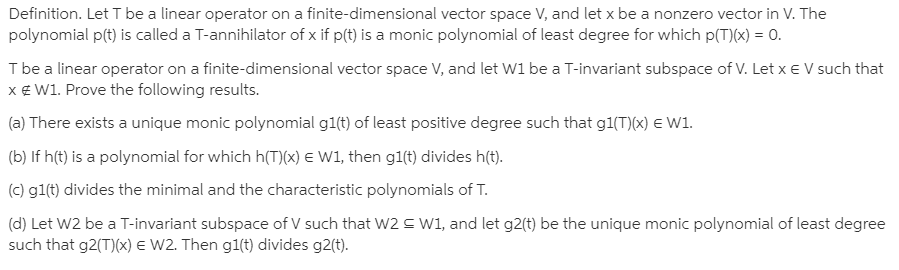# Definition. Let T be a linear operator on a finite-dimensional vector space V, and let x be a nonzero vector in V. Thepolynomial p(t) is called a T-annihilator of x if p(t) is a monic polynomial of least degree for which p(T)(x) = 0.I be a linear operator on a finite-dimensional vector space V, and let W1 be a T-invariant subspace of V. Let x e V such thatx€ W1. Prove the following results.(a) There exists a unique monic polynomial g1(t) of least positive degree such that g1(T)(x) E W1.(b) If h(t) is a polynomial for which h(T)(x) E W1, then g1(t) divides h(t).(c) gl(t) divides the minimal and the characteristic polynomials of T.(d) Let W2 be a T-invariant subspace of V such that W2 S W1, and let g2(t) be the unique monic polynomial of least degreesuch that g2(T)(x) E W2. Then g1(t) divides g2(t).

Question
2 viewshelp_outlineImage TranscriptioncloseDefinition. Let T be a linear operator on a finite-dimensional vector space V, and let x be a nonzero vector in V. The polynomial p(t) is called a T-annihilator of x if p(t) is a monic polynomial of least degree for which p(T)(x) = 0. I be a linear operator on a finite-dimensional vector space V, and let W1 be a T-invariant subspace of V. Let x e V such that x€ W1. Prove the following results. (a) There exists a unique monic polynomial g1(t) of least positive degree such that g1(T)(x) E W1. (b) If h(t) is a polynomial for which h(T)(x) E W1, then g1(t) divides h(t). (c) gl(t) divides the minimal and the characteristic polynomials of T. (d) Let W2 be a T-invariant subspace of V such that W2 S W1, and let g2(t) be the unique monic polynomial of least degree such that g2(T)(x) E W2. Then g1(t) divides g2(t). fullscreen

### This question hasn't been answered yet.